# Math behind SVM (Support Vector Machine)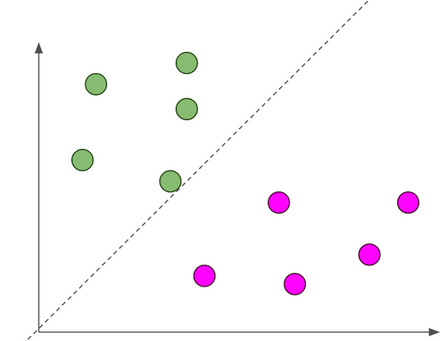dotted line is hyperplane, separating blue and pink classes balls.

## Vectors

Vectors are mathematical quantity which has both magnitude and direction. A point in the 2D plane can be represented as a vector between origin and the point.

## Length of Vectors

Length of vectors are also called as norms. It tells how far vectors are from the origin.

## Direction of vectors

Direction of vector .

## Dot Product

Dot product between two vectors is a scalar quantity . It tells how to vectors are related.

## Hyper-plane

It is plane that linearly divide the n-dimensional data points in two component. In case of 2D, hyperplane is line, in case of 3D it is plane.It is also called as n-dimensional line. Fig.3 shows, a blue line(hyperplane) linearly separates the data point in two components.

## What if data points is not linearly separable ?

Look Fig.4 how can we separate the data-points linearly? This type of situation comes very often in machine learning world as raw data are always non-linear here.So, Is it do-able? yes!!. we will add one extra dimension to the data points to make it separable.

## Margin and Support Vectors

Let’s assume that solid black line in above Fig.7 is optimal hyperplane and two dotted line is some hyperplane, which is passing through nearest data points to the optimal hyperplane. Then distance between hyperplane and optimal hyperplane is know as margin, and the closest data-points are known as support vectors. Margin is an area which do not contains any data points. There will be some cases when we have data points in margin area but right now we stick to margin as no data points lands.

1. Linear separable data points.
2. Linear separable data points II.
3. Non-linear separable data-points.

## Unconstrained optimization

Example will be more intuitive in explaining the concept,

## Constrained optimization

Again it will become clear with an example,

## Primal and Dual Concept

Shocked!! what the heck is this now???

## Optimization

This part will be more mathematical, some terms are very high level concept of mathematics, but don’t worry i will try to explain each one by one in layman term.

1. In first we will formulate SVM optimization problem Mathematically
2. we will find gradient with respect to learning parameters.
3. we will find the value of parameters which minimizes ||w||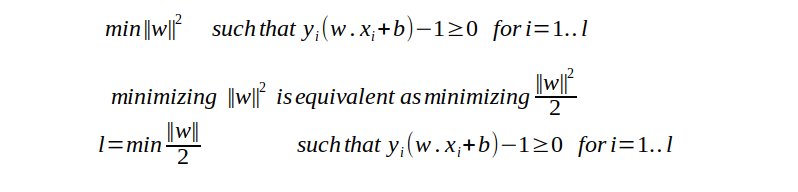PART I — Problem formulation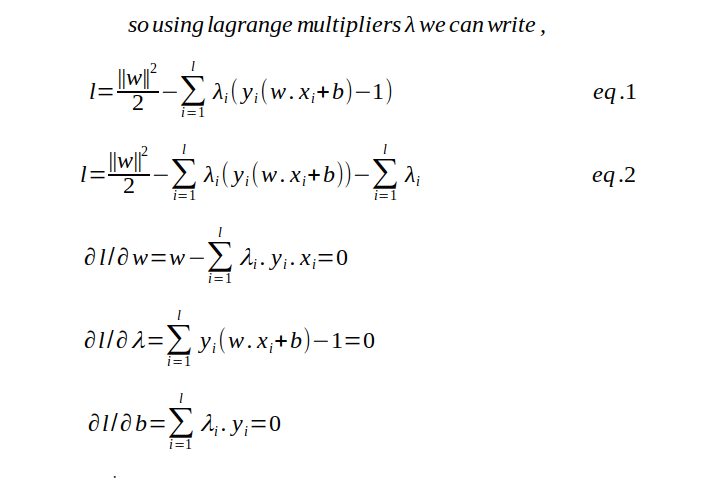PART II — — — finding the gradient with respect to w ,b and lambda.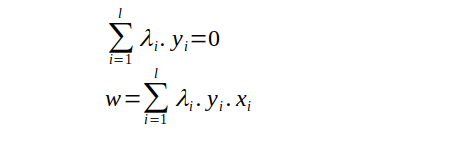PART III — — — — we will get the value of w
1. Problem formulation and substitution value from primal
2. Simplify the loss function equation after substitution
3. final optimization formulation to get the value of λ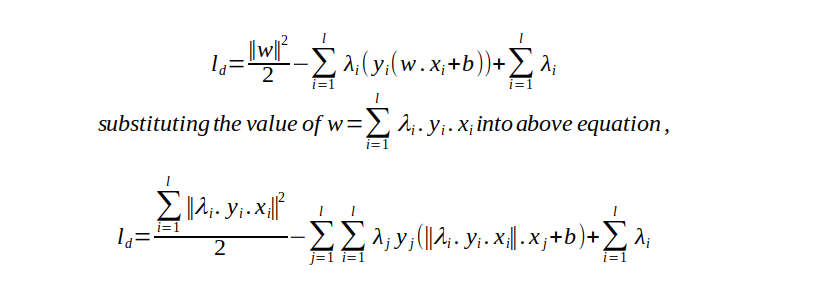PART I — — — — Formulation from primal and Substitution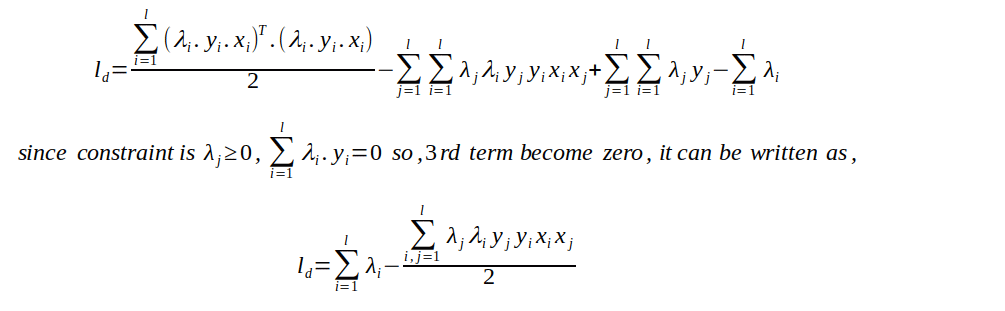PART II — — — — — — — — — SimplificationPART III — — — — — -Final optimization

w.x + b = 0

And a new example x_ can be classified as sign(w.x_ +b)

Thanks for reading, this is the PART1 of SVM, in PART 2 we will discuss about how to deal with a case when data is not fully linearly separable.

--

--